Mobile QR Code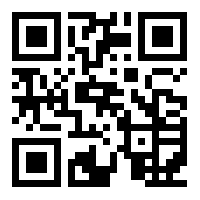1. (Basic Teaching Department, Hebei Women's Vocational College, Shijiazhuang, 050000, China)

Online sports course, Gradient descent method, Motion capture system, Data fusion

## 1. Introduction

The COVID-19 epidemic has greatly increased the difficulty of conducting offline physical education classes . Online physical education classes have attracted increasing attention to teach students physical education knowledge and ensure their health. On the other hand, online physical education class imparts knowledge through the Internet, and physical exercise movements cannot be taught well, resulting in poor learning effect for students . The inconvenience of online physical education teaching can be improved by embedding the human motion capture system into the online physical education course. The students’ learning effect can be improved by capturing the movements of teachers and students . The motion capture system generally solves and reconstructs the motion of the human body through the attitude calculation algorithm so that the human body model follows the movement of a real person . The current motion capture system is not accurate enough to capture movements, and it is difficult for the human body model to reproduce the movements of real people accurately. Therefore, it is important to explore a capture system that can capture human movements accurately. The gradient descent method is a common optimization algorithm that can be applied to improve the accuracy of the calculated attitude in attitude calculation . Therefore, the study integrates the gradient descent method into the human motion capture system, and then applies the system to the online physical education learning course to explore whether this method can improve the learning effect of online physical education teaching.

## 2. Literature Review

With the rapid development of electronic information technology, motion capture algorithms are being applied increasingly in various fields . Roberts et al. (2018) proposed to use curve fitting technology to interpolate the keyframes found to produce a new simplified animation, aiming at the problem that the motion capture algorithm is difficult to edit in games and movies. The algorithm could simplify motion capture to 10\% of the original frames . To improve the accuracy of small-scale human motion recognition in videos, the Gao team proposed a multi-dimensional data model for motion recognition and motion capture in video images based on a deep learning framework. The dataset and small gesture actions had high recognition accuracy . Li proposes a sensor body coordinate system calibration algorithm based on hand-eye calibration to capture dance movements better. The joint parameter estimation algorithm based on human joint constraints and the limb length estimation based on the closed joint chain was used. The results show that the model accurately recognizes human gestures . Aiming at the invasiveness of construction workers’ fatigue sensors, Yu et al. proposed a 3D motion capture algorithm based on computer vision. They used this algorithm to monitor the body fatigue of construction workers. After a series of experiments, they proved that the method could monitor the fatigue level of construction workers accurately under different conditions . The Liu team proposed a real-time interactive Taijiquan learning system based on virtual reality and motion capture technology to learn Taijiquan anytime and anywhere. They also compared the movements of students and virtual coaches using motion similarity matching algorithms to evaluate the quality of learning and training and guide learners to adjust their movements in real-time. Through empirical analysis, they found that the system could effectively help learners to carry out Taijiquan and learn and evaluate.

There are also various algorithms applied to the field of online teaching . Yu proposed an improved AprioriTid algorithm based on emotion recognition and data mining algorithms to evaluate the quality of online English online teaching accurately and used the model to conduct empirical analysis. The results show that the teaching quality evaluation model performs better than traditional models . Coincidentally, Fang proposed a teaching quality evaluation model integrating machine learning theory and principal component analysis to solve the problem of inaccurate evaluation of English online teaching quality. The model was applied to practice. The model was superior to the traditional teaching evaluation model in evaluating online teaching quality . To study the efficiency of the English online teaching system, Zhou constructed a model for improving the efficiency of English online teaching based on a machine learning algorithm and proposed a streaming feature selection algorithm based on bagging learning. The performance of the model was verified, and the constructed model for improving the efficiency of English online teaching had high reliability . Vivekanadam proposed a deep learning technology based on artificial intelligence to solve the problem of the low monitoring effect of online teaching courses. By testing the actual effect of this technology, they found that it could effectively monitor online teaching courses, greatly improving the quality of online courses.

From the above research, the motion capture algorithm has been successfully applied in various fields, and the methods applied to the online teaching field are also emerging, but research on the combination of the motion capture algorithm and online teaching is still relatively scarce. Therefore, the study applied the 3D motion capture algorithm to online physical education teaching based on data fusion to capture the movements of the students in an online physical education class to help carry out the online physical education class normally and efficiently. The students in online physical education classes could perform physical exercise better and improve their physical fitness.

## 3. Construction of the Motion Capture System based on Data Fusion and Construction of an Online Physical Education Teaching Model

### 3.1 Construction of the 3D Motion Capture System

After optimizing the 3D motion human capture algorithm, the 3D motion capture system should be constructed. The purpose of the research was to allow the objects of the online physical education course to wear inertial sensors so that the human body model could follow the students’ movements in real-time . In human motion capture, the number of inertial sensors and their wearing positions significantly impact the capture results. To capture human motion better, the number of inertial sensors was set to 15 by referring to the tree structure and considering the accuracy of the motion capture model. Fig. 1 shows their wearing positions in the human body.

As shown in Fig. 1, inertial sensors were worn at the pelvis, spine, head, left forearm, left forearm, left hand, right forearm, right forearm, right hand, left thigh, left calf, left foot, right thigh, right calf, and right foot of the human body, respectively. Human motion capture can be achieved by collecting information on these 15 positions. The data generation rate of the sensor was 100 Hz. The 3D motion capture system constructed by the research was divided into two parts: the upper computer and the lower computer. The lower computer was composed of inertial sensor nodes. Each node included an inertial sensor chip and a wireless module. The upper computer was a software platform. The data of the sensor nodes in the system was sent to the software platform for analysis and calculation. The model was then driven by the calculation results to realize the human body model following the action of the real person. The specific structural design of the 3D motion capture system is shown in Fig. 2.

##### Fig. 1. Wearing position of the human inertial sensor.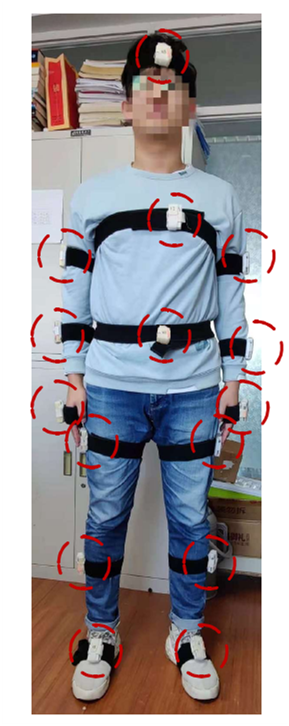##### Fig. 2. Overall design of the motion capture system.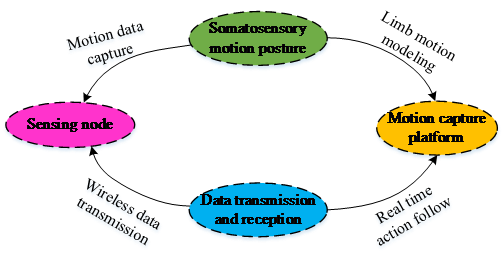##### Fig. 3. Schematic diagram of the algorithm structure.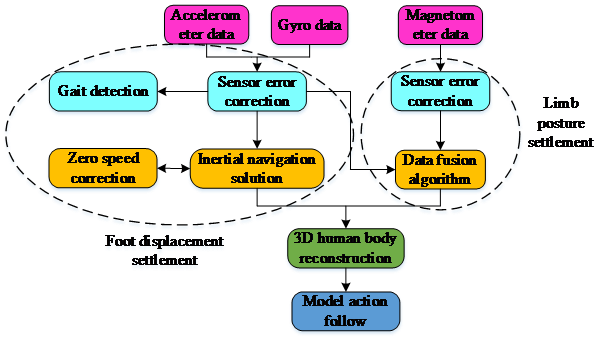As shown in Fig. 2, the 3D motion capture system was divided into four modules, a data acquisition module, a data transmission module, an action reconstruction module, and a data processing module. As the information input terminal of the system, the primary function of the data acquisition module was to collect and input the information on the inertial sensor worn on the human body. The information collected included the motion information on the gyroscope, accelerometer, and magnetometer on the sensor. All motion information was collected, organized, and packed. The wireless module was used to send it to the software. A crucial module in the motion capture system is the data transmission module, which ensures the integrity and speed of the transmitted information and the comfort and mobility of the body-worn sensor. Therefore, the data transmission method in this motion capture system is wireless transmission, and the received information data is transmitted to the upper computer software through the wireless module in each sensor node without affecting the information integrity and speed, improve the comfort of the human body. In addition to the above two modules, the data processing module of the motion capture system mainly includes three parts, which are data preprocessing, foot posture calculation, and each node posture calculation. The data preprocessing is mainly to correct the errors that may exist in the sensors, gyroscopes, and magnetometers; when the foot posture is calculated, and the posture of each node is calculated, the posture of the foot and each node of the human body was calculated to obtain the human body. The motion poses of the limbs were used for model reconstruction. As the last module of the motion capture system, motion reconstruction is one of the indicators to evaluate the quality of the motion capture system. In this module, the method of unconstrained nodes was used to reconstruct the human body posture, and the proposed human body model was used to test the accuracy of the reconstruction method. Finally, the 3D human body model was loaded on the software platform to follow the real human body in real time. Various algorithms were used in the capture system, and the specific algorithm structure is shown in Fig. 3.

The algorithm used in the capture system is divided into six parts: sensor error correction module, inertial navigation solution module, gait detection module, zero-speed correction module, data fusion algorithm module, and 3D human body Refactor module. Because the sensor has unavoidable errors in its process, and these errors have a significant influence on the results, it is necessary to correct the data transmitted by the sensor. The sensor error correction module is mainly to correct the received raw data. (Ed note: Get'' should not be used in written English. Other synonyms should be chosen.) Obtaining more accurate data makes the overall model more accurate. The primary function of the inertial navigation calculation module is to calculate foot displacement. It updates the formulae of human posture, speed, and position by working with the zero-speed correction algorithm and error correction. It solves the position of the human foot in real time. The gait detection module distinguishes the support phase and the swing phase to facilitate the subsequent zero-speed correction calculation. The zero-speed correction module suppresses the navigation error in the support phase and improves the accuracy of the foot displacement result. The core algorithm in the motion capture system is the data fusion algorithm module. In this module, the data fusion algorithm is used to solve the collected gyroscope, accelerometer, and magnetometer data information to obtain the key posture data of the human body. After obtaining the key posture data of the human body, the human body movement posture is tracked in the 3D human body reconstruction module, and the position of the root node is estimated by using the foot space position in this module, to realize the model following the human body movement. In the process of online physical education, the motion capture system can be used to capture the teaching actions of the teacher and the learning actions of the students in class, and the actions of the students can be compared with the actions of the teacher, to evaluate the learning quality of the students. (Ed note: Short coordinating conjunctions like and'' and but'' should not be used at the beginning of sentences.)Moreover, it can find students’ problems quickly and help them improve their movements.

### 3.2 3D Action Body Capture based on Data Fusion

The human body model plays a key role in human motion capture. The human body model will change with posture. Its posture update is based on a node of its tree structure as the root node. The attitude algorithm will be used to update the pose of the human model. A good attitude algorithm greatly impacts the accuracy of human motion capture. Because the gradient descent method can find the optimal solution of the objective function, it can help the human body model to find the optimal solution better in the pose solution process and help improve the accuracy of human motion capture. The gradient descent method has a simple structure and produces stable and reliable results . Therefore, this paper proposes a method of attitude calculation based on the gradient descent method, which is used to capture human motion. In this solution method, the attitude error is represented by an error function. When used for 3D motion human body capture, the error is generated mainly by the accelerometer and magnetometer. In the process of human posture recognition, the human posture is expressed by a quaternion, and its expression is $q=q_{0}+q_{1}i+q_{2}j+q_{3}k$, where $q_{0}$, $q_{1}$, $q_{2}$, and $q_{3}$ are real numbers. The error caused by the acceleration in the proposed method is $F_{f}(q,f)$, and its expression is shown in Eq. (1).

##### (1)
$F_f(q, f)=\left[\begin{array}{c} \frac{\partial F_{f x}}{d q} \\ \frac{\partial F_{f y}}{d q} \\ \frac{\partial F_{f z}}{d q} \end{array}\right]=\left[\begin{array}{c} 2\left(q_1 q_3-q_0 q_2\right)-f_x^b \\ 2\left(q_0 q_1-q_2 q_3\right)-f_y^b \\ 2\left(\frac{1}{2}-q_1^2-q_2^2\right)-f_x^b \end{array}\right]$

Eq. (1), $f_{x}^{b}$, $f_{y}^{b}$, and $f_{z}^{b}$are the measured values of the accelerometer in three directions in the carrier coordinate system. The carrier coordinate system is connected to the carrier, and the coordinate origin is the coordinate system of the center of the carrier, referred to as the b system. In Eq. (1), $b$ represents the carrier coordinate system, $q$ represents the quaternion of human posture, and $f$ represents the measured value. The error generated by the magnetometer is used to express that(Ed note: I am unclear what that'' is. The estimation vector of the magnetometer needs to be expressed first to calculate the magnetometer error. The navigation-coordinate system is the reference coordinate system used to determine the navigation parameters of the carrier, referred to as the n-system. In the navigation-coordinate system, the expression of the estimated vector of the magnetometer is shown in Eq. (2).

##### (2)
$h=\left(\begin{array}{lll} h_{x} & h_{y} & h_{z} \end{array}\right)^{T}=C_{b}^{n}\frac{m^{b}}{\left\| m^{b}\right\| }$

where $h_{x}$, $h_{y}$and $h_{z}$are the measured values of the magnetometer in three directions in the navigation-coordinate system, and $m^{b}$are the observation vectors in the carrier coordinate system, $n$represents the navigation-coordinate system. Among them $h_{x}=\sqrt{(m_{x}^{n})^{2}+m_{y}^{n})^{2}}$, $h_{y}=0$, $h_{z}=m_{z}^{n}$, will be $h$transformed into the estimated vector in the carrier-coordinate system, $h'$, and its expression is shown in Eq. (3).

##### (3)
$h^{\prime}=C_n^b\left[\begin{array}{c} h_x \\ 0 \\ h_z \end{array}\right]=\left[\begin{array}{c} 2 h_x\left(\frac{1}{2}-q_2^2-q_3^2\right)+2 h_z\left(q_1 q_3-q_0 q\right) \\ 2 h_x\left(q_1 q_2-q_0 q_3\right)+2 h_z\left(q_0 q_1+q_1 q_3\right) \\ 2 h_x\left(q_0 q_2+q_1 q_3\right)+2 h_z\left(\frac{1}{2}-q_1^2-q_2^2\right) \end{array}\right]$

The expression of magnetometer error $F_{m}(q,m)$ is shown in Eq. (4).

##### (4)
$F_{m}\left(q,m\right)=\left[\begin{array}{l} \frac{\partial F_{mx}}{dq}\\ \frac{\partial F_{my}}{dq}\\ \frac{\partial F_{mz}}{dq} \end{array}\right]=\left[\begin{array}{l} 2h_{x}\left(\frac{1}{2}-q_{2}^{2}-q_{3}^{2}\right)+2h_{z}\left(q_{1}q_{3}-q_{0}q\right)-m_{x}^{b}\\ 2h_{x}\left(q_{1}q_{2}-q_{0}q_{3}\right)+2h_{z}\left(q_{0}q_{1}+q_{1}q_{3}\right)-m_{y}^{b}\\ 2h_{x}\left(q_{0}q_{2}+q_{1}q_{3}\right)+2h_{z}\left(\frac{1}{2}-q_{1}^{2}-q_{2}^{2}\right)-m_{z}^{b} \end{array}\right]$

Eq. (4), $\frac{\partial F_{mx}}{dq}$, $\frac{\partial F_{my}}{dq}$, and $\frac{\partial F_{mz}}{dq}$, respectively, represent the errors of the magnetometer in three directions in the carrier coordinate system. The expression of the total error can be obtained by combining the errors of the magnetometer and the acceleration, as shown in Eq. (5).

##### (5)
$F_{f,m}\left(q,f,m\right)=\left[\begin{array}{l} F_{f}\left(q,f\right)\\ F_{m}\left(q,m\right) \end{array}\right]$

The partial derivative of Eq. (5) was calculated to obtain the expression shown in Eq. (6).

##### (6)
$\begin{array}{l} J_{f,m}\left(q,f,m\right)=\left[\begin{array}{l} J_{f}\left(q,f\right)\\ J_{m}\left(q,m\right) \end{array}\right]\\ =\left[\begin{array}{llll} -2q_{2} & 2q_{3} & -2q_{0} & 2q_{1}\\ 2q_{1} & 2q_{0} & 2q_{3} & 2q_{2}\\ 0 & -4q_{1} & -4q_{2} & 0\\ \begin{array}{l} -2h_{z}q_{2}\\ \begin{array}{l} -2h_{x}q_{2}+2h_{z}q_{1}\\ 2h_{x}q_{2} \end{array} \end{array} & \begin{array}{l} 2h_{z}q_{3}\\ 2h_{x}q_{2}+2h_{z}q_{0}\\ 2h_{x}q_{3}-4h_{z}q_{1} \end{array} & \begin{array}{l} -4h_{x}q_{2}-2h_{z}q_{0}\\ 2h_{x}q_{1}+2h_{z}q_{3}\\ 2h_{x}q_{0}-4h_{z}q_{2} \end{array} & \begin{array}{l} -4h_{x}q_{3}+2h_{z}q_{1}\\ -2h_{x}q_{0}+2h_{z}q_{2}\\ 2h_{x}q_{1} \end{array} \end{array}\right] \end{array}$

To minimize the error of Eq. (6), it(Ed note: I am unsure what it'' is.) was calculated using Gauss\textendash{}Newton method, and $q_{\nabla ,t}$the calculation target is expressed as\_(ed note: What is it expressed as? Please check the change.) Eq. (7).

##### (7)
$q_{\nabla ,t}=q_{\nabla ,t-1}-\mu \frac{\nabla F_{f,m}\left(q,f,m\right)}{\left\| \nabla F_{f,m}\left(q,f,m\right)\right\| }$

where $\mu$ is the step size; $\nabla$ is a differential operator; $\nabla F_{f,m}(q,f,m)$ expressed as Eq. (8).

##### (8)
$\nabla F_{f,m}\left(q,f,m\right)=J_{f,m}^{T}\left(q\right)F_{f,m}\left(q,f,m\right)$

The gradient-descent data-fusion algorithm is used to solve the attitude; the algorithm flow chart is shown in Fig. 3.

The algorithm flow chart in Fig. 4 shows that the errors obtained from the magnetometer and accelerometer are passed through the Jacobian matrix of the error function to obtain the gyroscope correction value. The differential equation was then solved through the gyroscope output to obtain the final attitude quaternion. Eq. (9) shows the expression of the final attitude quaternion.

##### (9)
$q_{t}=\alpha q_{\nabla ,t}+\left(1-\alpha \right)q_{\omega ,t}$

where $q_{t}$ represents the final attitude quaternion; $\alpha$ is the weight of the corrected quaternion; $q_{\omega ,t}$ is the quaternion obtained by solving the differential equation. If $\beta$ is the gyroscope error and $t_{s}$ the sampling period, Eq. (10) can be used.

##### (10)
$\left(1-\alpha \right)\beta =\alpha \frac{\mu }{t_{s}}$

where $\alpha$ is the weight, and $\mu$ is the step size. In this case, $q_{t}$, the expression can be changed to Eq. (11).

##### (11)
$q_{t}=q_{t-1}+\left(q_{t-1}-\beta \frac{\nabla F_{f,m}\left(q,f,m\right)}{\left\| \nabla F_{f,m}\left(q,f,m\right)\right\| }\right)t_{s}$

Initialization and calibration are very important processes in human motion capture. They solve the rotation quaternion from the carrier coordinate system to the sensor coordinate system. The content of the motion capture is the motion posture of the human body. The content of the motion capture is also inaccurate. When the initial calibration is performed, the carrier coordinates coincide with the geographic coordinate system. The geographic coordinate system takes the position of the carrier as the origin of the coordinate system. Generally, the attitude description of the carrier is roll angle, pitch angle, and yaw angle. At this time, the expression is shown in Eq. (12).

##### (12)
$q_s^n\left(t_i\right)=q_s^b\left(t_i\right)$

where $t_{i}$ is the initial state. The axis of the sensor coordinate system of the inertial sensor uses the coordinate axis constrained by the three sensitive axes of the gyroscope to form a right-hand rectangular coordinate system that unifies the coordinate axes of other inertial measuring elements into the sensor coordinate system, which is referred to as the s system. The transformation relationship between the sensor coordinate system and the carrier coordinate system is shown in Eq. (13).

##### (13)
$q_s^b\left(t_i\right)=q_s^b(t)$

where $s$ is the sensor coordinate system and $b$ is the carrier coordinate system. The purpose of this system is to make the human body model follow the movement of the human body in reality, solve the posture quaternion of each moving limb in the navigation-coordinate system to the rotation quaternion between two connected limbs, and solve the joints in the human body model. Solving the three-dimensional coordinates of the points is the focus of the research. Taking the moving limbs of the lower limbs of the human body as an example, the solution process is explained. Fig. 5 shows the structure diagram of the lower limbs of the human body.

##### Fig. 4. Flow chart of the gradient descent algorithm.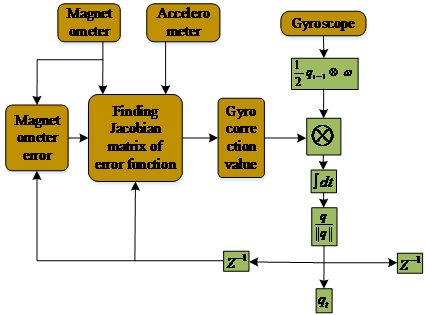##### Fig. 5. Structural diagram of the human lower limbs.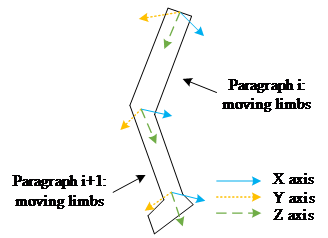##### Fig. 6. Node traversal update sequence.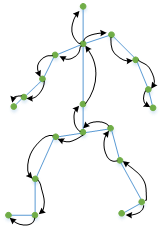As shown in Fig. 5, $i$ the quaternion of rotation between the two moving limbs can be represented $q_{i}^{i+1}$ by $i+1$ denoting the first $i+1$ segment of the moving limb, and the first segment of the moving limb $i$. In addition, $i$ the rotation quaternion of the first limb sensor coordinate system relative to the navigation-coordinate system is used ${ }_s^n q_i$${ }_b^s q_i$ to represent the rotation matrix of the first $i$limb relative to the wearable sensor coordinate system. The quaternion from the navigation-coordinate system to the carrier coordinate system can be obtained through the sum of ${ }_b^n q_i$, ${ }_s^n q_i$, and ${ }_b^s q_i$; its expression is shown in Eq. (14).

##### (14)
${ }_b^n q_i={ }_s^n q_i \otimes{ }_b^s q_i$

A similar ${ }_b^n q_{i+1}$ expression is shown in Eq. (15).

##### (15)
${ }_b^n q_{i+1}={ }_s^n q_{i+1} \otimes{ }_b^s q_{i+1}$

where ${ }_b^n q_{i+1}$ is the $i+1$ rotation matrix of the first limb relative to the coordinate system of the wearable sensor. Eq. (16) can be obtained using Eqs. (14) and (15).

##### (16)
${ }^b q_i^{i+1}={ }_n^b q_{i+1} \otimes{ }_n^b q_i$

where ${ }^b q_i^{i+1}$ represents the rotation quaternion expression between two moving limbs. In space, coordinate transformation can be accomplished through coordinate translation, and its transformation equation is shown in Eq. (17).

##### (17)
$\left[\begin{array}{l} x\\ y\\ z \end{array}\right]=\left[C\right]\left[\begin{array}{l} x_{0}\\ y_{0}\\ z_{0} \end{array}\right]+\left[T\right]$

where the $\left[x_{0},y_{0},z_{0}\right]$ original coordinates are $\left[x,y,z\right]$, and the converted coordinates are represented. $C$ is a left-multiplying rotation matrix, and $T$ is a translation vector. The human body tree model can represent the real human body in the motion capture system. The positions of its joints determine the spatial position of the human body model. Therefore, if the spatial position of a certain limb is known, the posture and position of the human body model following the movement of the human body can be inferred. For the motion capture system, the most likely cause of the error is its drift problem. Selecting the pelvis position as the root node can improve the drift problem, but this method will ignore the movement of the human body in space, such as jumping. The squatting action cannot be tracked effectively . The research adopted the method of unconstrained root to solve the problem of not being able to track the ups and downs of the human body correctly due to the selection of the pelvis as the root node and used the spatial position of the foot to solve the drift problem . The foot space position was calculated using the zero-speed update algorithm. The calculation result was accurate, and the drift error was small. Fig. 6 presents the traversal sequence of the human body nodes.

As shown in Fig. 6, the human body takes the foot as the starting point for traversal calculation, obtains the position of the pelvic root node from the foot through the lower limb traversal, and then recurses the spatial position of other nodes through the tree structure model of the human body to realize Action refactoring. When the zero-speed update algorithm calculates the foot displacement, the foot state is divided into support and swing phases. When the foot is in the swing phase, the inertial navigation algorithm can be used to calculate the foot state. When the foot is in the support phase, the foot error can be estimated and corrected, and the accurate foot space position can be obtained by combining the two methods. After obtaining the accurate foot space position, the pose of the human body can be reconstructed using the root-free method. Similar algorithms for human motion capture include the human motion real-time tracking strategy proposed by Pengzhan et al. in 2016. This method captures the posture of human motion through the complementary and Kalman filters with high accuracy . Compared with the tracking strategy proposed in the study, the root unconstrained method is added, which can be traversed from the foot as the starting point. This method has a higher accuracy rate for capturing the motion posture with supporting phase and short swing time and is more suitable for online sports teaching.

## 4. Performance Verification of Attitude Calculation Algorithm and Empirical Analysis of Motion Capture System

### 4.1 Performance Verification of Data Fusion Attitude Solution Algorithm

The Euler angle and quaternion methods in the data calculation algorithm generally represent the attitude. The Euler angle method has a better visualization effect when expressing the attitude. Therefore, to verify the performance of the data-fusion attitude calculation algorithm, the Euler angle was used as the index of the attitude calculation algorithm, and the data-fusion attitude calculation algorithm and Kalman filtering algorithm proposed by Zhao et al. were compared to verify the performance . The sensors corresponding to the two attitude calculation algorithms were worn on the same sensor nodes on the hand, and two actions were performed to compare the performance of the two algorithms. The first step in the first action was to keep the palm in a horizontal state. The second step was to bend the wrist down and then return to the horizontal state. In the third step, the wrist was bent up and then returned to the horizontal state; the next two steps were followed. These three steps are then repeated.

The second action was like the first step. The second step was to bend the wrist upwards and then return to a horizontal state, and the third step was to bend the wrist downwards and then return to a horizontal state; the last two steps were repeated. Fig. 7 shows the action pose curves of the two algorithms.

Fig. 7 shows that the action posture curve of the data-fusion posture solution algorithm is close to the actual action posture curve and far superior to the Kalman filter algorithm. The posture curve shows that the data-fusion algorithm can compensate for the shortcomings of the sensor, improve the anti-interference ability of the sensor, and accurately collect the correct motion posture to achieve accurate motion capture. In addition to using Euler angles to compare the performance of the algorithms, the research also uses limb-tracking accuracy as the performance index of the algorithm. It uses the joint angles to represent its limb-tracking accuracy. Sensors corresponding to the two algorithms were worn in appropriate positions, and the following exercises were performed. The first step was to let the arm hang down naturally. The second step was to raise the left forearm to 90$^{\circ}$, and the third step was to place the left forearm back in the initial state. The three-step exercise was then repeated. Fig. 8 shows the joint angle change curves of the two algorithms.

Fig. 8 shows that the joint angle change curve of the data-fusion attitude calculation algorithm is very close to the actual curve. In contrast, the Kalman filter algorithm is quite different from the actual angle change curve. The data for arm raising and lowering were recorded four times, and the errors of the four repeats of the data-fusion attitude calculation algorithm were 1.67$^{\circ}$, 2.35$^{\circ}$, 1.38$^{\circ}$, and 1.89$^{\circ}$, respectively. The results show that the performance of the data fusion attitude solution algorithm is better than that of the Kalman filter algorithm. It can meet the accuracy requirements of 3D human motion capture.

##### Fig. 7. Action posture curves of the two algorithms.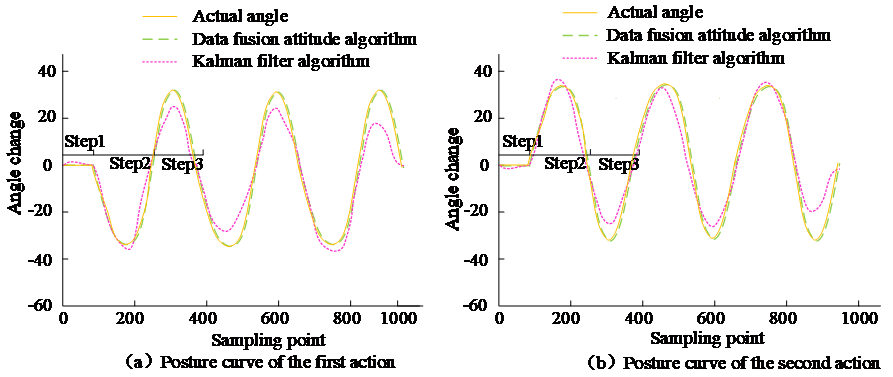##### Fig. 8. Joint angle curve of the two algorithms.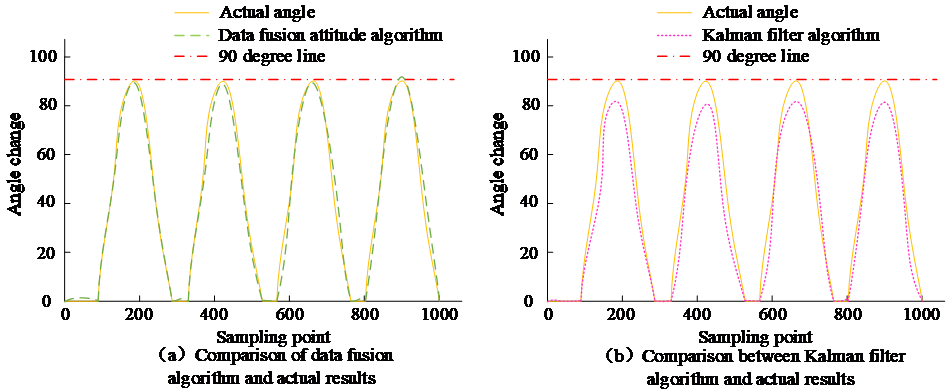### 4.2 Empirical Analysis of Motion Capture System and Online Physical Education Learning Software

To verify that the proposed 3D human motion capture system can drive the human body model to follow the real person correctly and can capture the position of the human body accurately through the position of the root node. The study carried out the 8'' path walking experiment on the motion capture system. Fig. 9 shows the results of the 8'' path-walking experiment.

The motion trajectory of the pelvic plane of the human body model of the motion capture system was similar to the actual walking motion trajectory (Fig. 9), indicating that the motion capture system can correctly capture the trajectory of the 8'' path walking. In the 8'' path walking experiment, the pelvic position and heading change curves were also obtained, as shown in Fig. 10.

##### Fig. 9. Results of 8'' path walking experiment.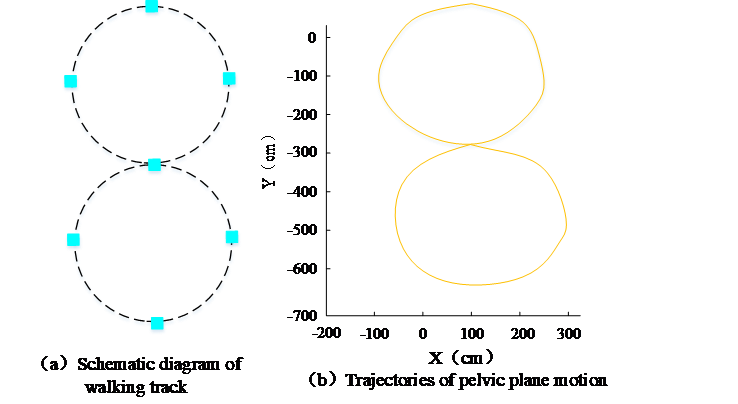##### Fig. 10. Pelvic position and course change curve.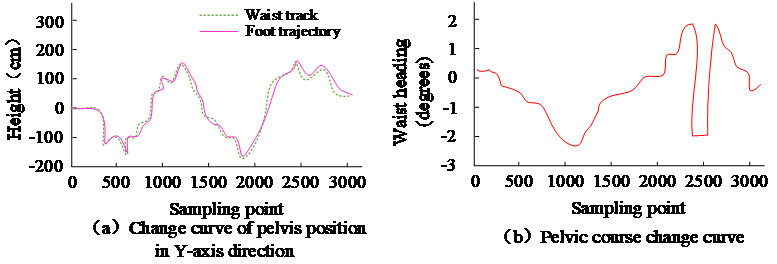The position change curves of the pelvis and feet of the human body model in the Y-axis direction were roughly the same, and the position changed basically conformed to the 8'' path trajectory (Fig. 10(a)). The change of the pelvic heading also basically conformed to the 8'' path trajectory(Fig. 10(b)). These results show that the position of the human body can be captured accurately by the position of the root node. The human body model controlled by the motion capture system can also capture the walking trajectory of the 8'' path of a real person accurately. In addition to the performance verification of the motion capture system, the satisfaction, learning efficiency, teaching efficiency, and learning effect of the online physical education learning course composed of the motion capture system were also compared with those of traditional online physical education learning courses and traditional online physical education courses based on traditional actions. The online physical education courses of the capture system were compared, as shown in Table 1. Satisfaction, learning efficiency, teaching efficiency, and learning effect are on a percentile scale. The higher the score, the better the effect.

As shown in Table 1, the satisfaction, learning efficiency, teaching efficiency, and learning effect of the online physical education course composed of the motion capture system based on the research design were superior to those of the other two online sports in the two groups of research objects. Among them, the average satisfaction, average learning efficiency, average teaching efficiency, and average learning effect of the online physical education course composed of the motion capture system based on the research design were 90.65, 91.15, 92.2, and 92.75, respectively. The online physical education learning course composed of the motion capture system designed in the present study is more popular with students than the other two online physical education courses, and it has a better effect on teachers’ teaching and students’ learning.

##### Table 1. Comparison of three online physical education courses.
 / Online physical education course based on motion capture system designed by research Traditional online physical education courses Online physical education learning course based on traditional motion capture system Group 1 satisfaction 90.2 75.8 80.1 Group 1 learning efficiency 91.3 77.2 83.8 Group 1 teaching efficiency 92.1 76.7 85.3 Group 1 learning effect 92.5 75.1 80.6 Group 2 satisfaction 91.1 77.6 81.5 Group 2 learning efficiency 90.8 75.5 82.7 Group 2 teaching efficiency 92.3 75.4 84.2 Group 2 learning effect 93.0 74.2 79.9

## 5. Conclusion

Given the inconvenient teaching of physical movements in online physical education courses, this research integrated the gradient descent method into attitude calculation to improve the accuracy of attitude calculation, embedded the improved attitude calculation algorithm into the human motion capture system, and applied the online motion capture system to physical education courses to improve the teaching quality of online physical education courses. The comparison experiment of attitude calculation performance showed that the improved attitude calculation algorithm has higher capture accuracy and an error angle of 1.67$^{\circ}$, which is far superior to the Kalman filter algorithm. In addition, the empirical analysis of the capture system and online sports courses showed that the motion capture system could accurately capture the position of the human body through the position of the root node. The satisfaction, learning efficiency, teaching efficiency scores, and learning effect are 90.65, 91.15, 92.2, and 92.75, respectively, which are better than the other two online physical education courses. These results show that the improved attitude calculation algorithm can effectively improve the teaching quality of online physical education courses. but

The range of sensing nodes of the motion capture system designed by the research was relatively narrow, and there was no motion capture for fingers. The follow-up research direction was to capture human gestures accurately.

### REFERENCES

1
Jeon. SM. An autoethnography on the adaptation online and offline classes of a physical education specialist in elementary schools,'' The Korean Journal of the Elementary Physical Education, vol. 26, no. 3, pp. 29-42, 2020.2
Chan. SY, Kim. HS. Relationship among high school students'' trust in teachers, interactions and learning satisfaction in online physical education classes caused by COVID-19,'' Journal of Korean Society for the Study of Physical Education, vol. 25, no. 4, pp. 123-134, 2021.3
Gao. P, Zhao. D, Chen. X. Multi-dimensional data modelling of video image action recognition and motion capture in deep learning framework,'' IET Image Processing, vol. 14, no. 7, pp. 1257-1264, 2020.4
Nian. Q, Liu. J. Motion capture based on intelligent sensor in snow and ice sports,'' Wireless Communications and Mobile Computing, no. 12, pp. 1-12, 20215
Wang. Y, He. Y, Zhu. Z. Study on fast speed fractional order gradient descent method and its application in neural networks,'' Neurocomputing, no. 489, pp. 366-376, 2022.6
Roberts. R, Lewis. JP, Anjyo. K, Seo. J, Seol. Y. Optimal and interactive keyframe selection for motion capture,'' Computational Visual Media, vol. 5, no. 2, pp. 171-191, 2018.7
Gao. P, Zhao. D, Chen. X. Multi-dimensional data modelling of video image action recognition and motion capture in deep learning framework,'' IET Image Processing, vol. 14, no. 7, pp. 1257-1264, 2020.8
Li. Y. Dance motion capture based on data fusion algorithm and wearable sensor network,'' Complexity, vol. 2021, no. 1, pp. 1-11, 2021.9
Yu. Y, Li. H, Yang. X, Kong. L, Wong. AY. An automatic and non-invasive physical fatigue assessment method for construction workers,'' Automation in Construction, no. 103, pp. 1-12, 2019.10
Liu. J, Zheng Y, Wang. K, et al. A real-time interactive Tai Chi learning system based on VR and motion capture technology,'' Procedia Computer Science, no. 174, pp. 712-719, 2020.11
Yu. H. Online teaching quality evaluation based on emotion recognition and improved AprioriTid algorithm,'' Journal of Intelligent and Fuzzy Systems, vol. 40, no. 5, pp. 1-11, 2020.12
Fang. C. Intelligent online teaching system based on SVM algorithm and complex Network,'' Journal of Intelligent and Fuzzy Systems, vol. 40, no. 5, pp. 1-11, 2020.13
Zhou. F. Efficiency improvement of English online teaching system based on bagging learning flow feature selection,'' Journal of Intelligent and Fuzzy Systems, no. 3, pp. 1-11, 2020.14
Vivekanadam. B. Evaluation of activity monitoring algorithm based on smart approaches,'' Journal of Electronics and Informatics, vol. 2, no. 3, pp. 175-181, 2020.15
Papapicco. V, Chen. B, Munih. M, Davalli. A, Sacchetti. R, Gruppioni. E, Vitiello. N. A classification approach based on directed acyclic graph to predict locomotion activities with one inertial sensor on the thigh,'' IEEE Transactions on Medical Robotics and Bionics, vol. 3, no. 2, pp. 436-445, 2021.16
Lu. L, Zheng. Z, Champagne. B, Yang. X, Wu. W. Self-regularized nonlinear diffusion algorithm based on Levenberg gradient descent,'' Signal Processing, no. 163, pp. 107-114, 2019.17
Tong. L, Liu. R, Peng. L. LSTM-based lower limbs motion reconstruction using low-dimensional input of inertial motion capture system,'' IEEE Sensors Journal, vol. 20, no. 7, pp. 3667-3677, 2019.18
V. Arufe-Giráldez, Cristobal. RV, Folgar. MI. Can a didactic proposal for the Physical Education classroom inspired by the Fortnite video game develop the values and various psychological variables in children and adolescents?'' Sport Science, vol. 14, no. 1, pp. 68-78, 2021.19
Pengzhan. C, Ye. K, Jie. L. Human motion capture algorithm based on inertial sensors,'' Journal of Sensors, no. 7, pp. 1-15, 2016.20
Zhao. YX, Hsieh. YZ, Lin. SS, Pan. CJ, Nan. CW. Design of an IoT-based mountaineering team management device using Kalman filter algorithm,'' Journal of Internet Technology, vol. 21, no. 7, pp. 2085-2093, 2021.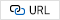## Author

Weiguo Li, male, was born in September, 1980. His title is associate professor. He graduated from Institute of Physical Education Hebei Normal University in 2005, major in Physical Education, with a baccalaureate. He graduated from Institute of Physical Education Hebei Normal University in 2008, major in Theory of Sports Pedagogy and Training，with a master's degree. Now he is working in Basic Teaching Department, Hebei Women's Vocational College. He mainly engages in the research of Physical education and training. So far, He has published more than 20 academic papers, participated in more than 10 scientific research projects, and published 3 sports textbooks.

Yongli Yang, female, Hebei women's vocational and technical college, lecturer, Her research interests include physical education, sports training, sports culture, etc. She has published 2 papers, presided over 1 university level and participated in 3 university level scientific research projects, a book was published.

Jing Zhou, female, Hebei women's vocational and technical college, lecturer, Her research interests include physical education, sports training, sports culture, etc. She has published 1 paper, participated in 4 scientific research projects above university level, published 1 book.

Zhipeng Li, male, lecturer, Hebei Women's Vocational College. His research interests include physical education teaching, sports training, sports culture, etc. He has published more than 5 papers, presided over 4 research projects at or above the university level and department level, and published 2 works and books.